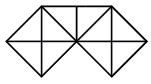Question 1

# How many triangles are there in the following figure?Solution

Each square shape has total of 8 triangles.

2 square's contain total of 16 triangles.

Other part than Square has 3 triangles and that part joined to 2 squares has 2 large triangles.

So, total number of triangles $$=16+3+2=21.$$

B is correct choice.